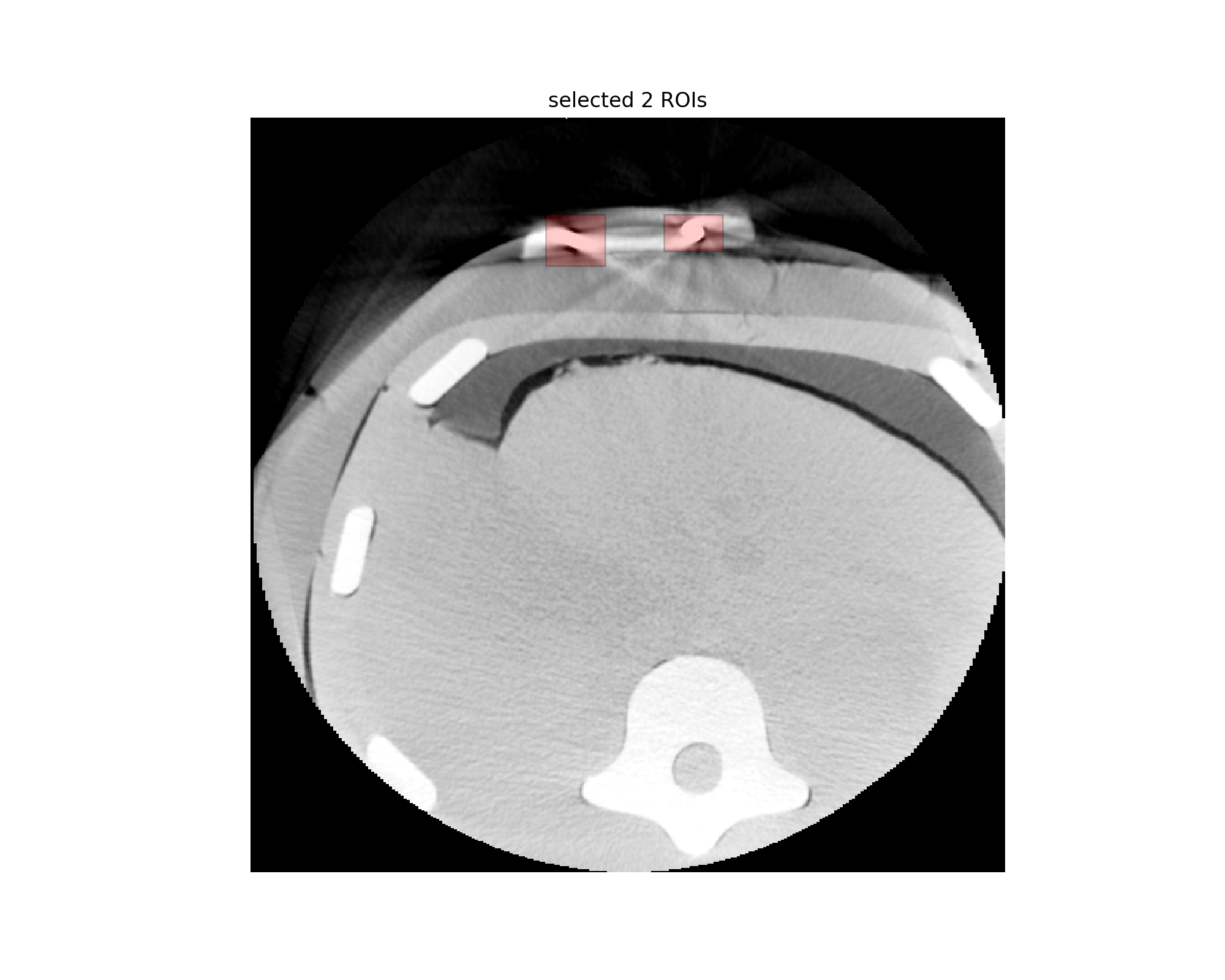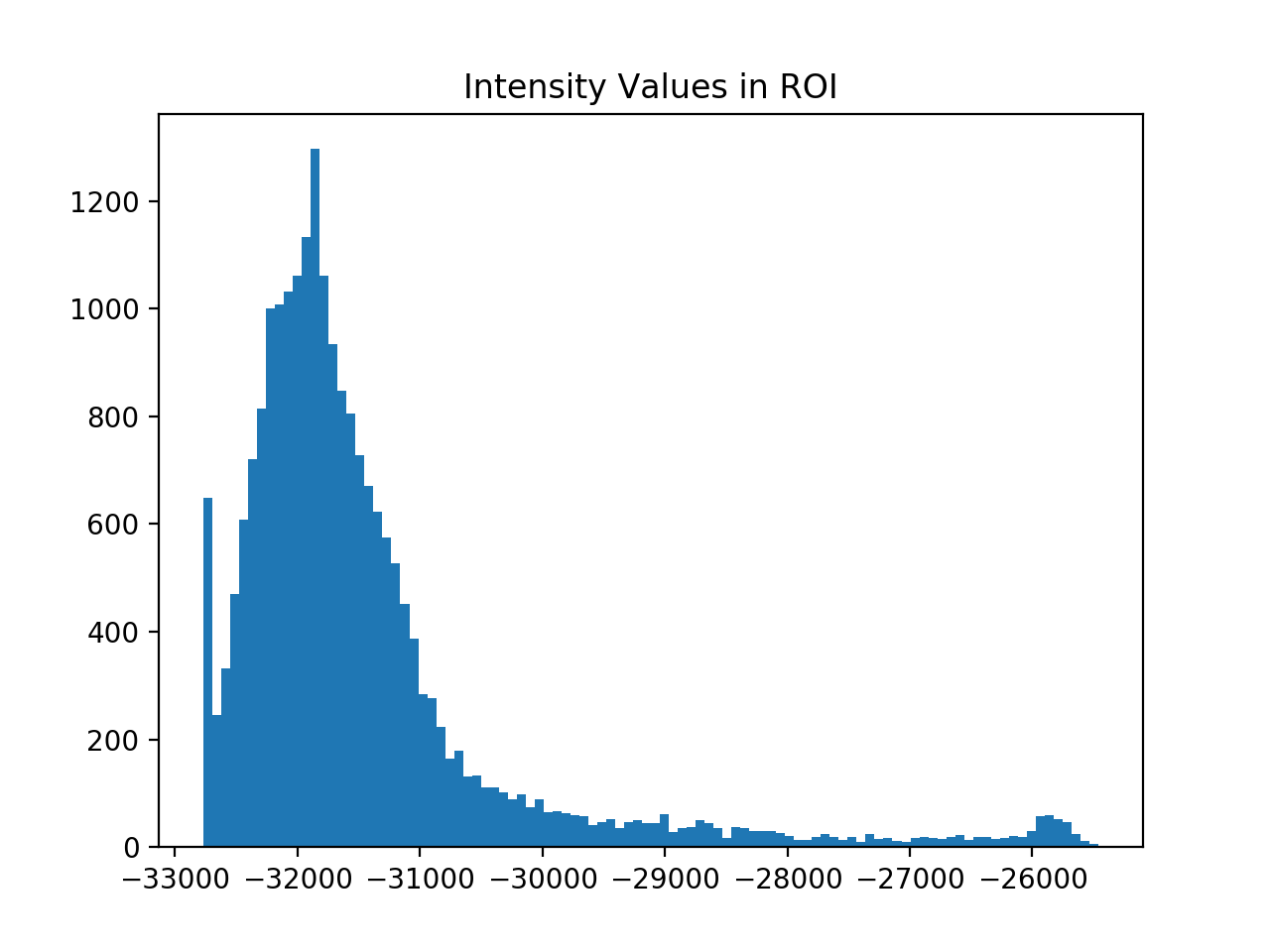# Segmentation: Thresholding and Edge Detection

In this notebook our goal is to estimate the location and radius of spherical markers visible in a Cone-Beam CT volume.

We will use two approaches:

1. Segment the fiducial using a thresholding approach, derive the sphere's radius from the segmentation. This approach is solely based on SimpleITK.
2. Localize the fiducial's edges using the Canny edge detector and then fit a sphere to these edges using a least squares approach. This approach is a combination of SimpleITK and scipy/numpy.

Note that all of the operations, filtering and computations, are natively in 3D. This is the "magic" of ITK and SimpleITK at work.

The practical need for localizing spherical fiducials in CBCT images and additional algorithmic details are described in: Z. Yaniv, "Localizing spherical fiducials in C-arm based cone-beam CT", Med. Phys., Vol. 36(11), pp. 4957-4966.

In :
import SimpleITK as sitk

%matplotlib notebook
import gui
import matplotlib.pyplot as plt

import numpy as np
from scipy import linalg

from ipywidgets import interact, fixed


Load the volume, it contains two spheres. You can either identify the regions of interest (ROIs) yourself or use the predefined rectangular regions of interest specified below ((min_x,max_x), (min_y, max_y), (min_z, max_z)).

To evaluate the sensitivity of the algorithms to the image content (varying size and shape of the ROI) you should identify the ROIs yourself.

In :
spherical_fiducials_image = sitk.ReadImage(fdata("spherical_fiducials.mha"))
roi_list = [((280,320), (65,90), (8, 30)),
((200,240), (65,100), (15, 40))]

Fetching spherical_fiducials.mha


We use a GUI to specify a region of interest. The GUI below allows you to specify a box shaped ROI. Draw a rectangle on the image (move and resize it) and specify the z range of the box using the range slider. You can then view the ROI overlaid onto the slices using the slice slider. The toolbar on the bottom of the figure allows you to zoom and pan. In zoom/pan mode the rectangle interaction is disabled. Once you exit zoom/pan mode (click the button again) you can specify a rectangle and interact with it.

We already specify two ROIs containing the two spheres found in the data (second row below).

To evaluate the sensitivity of the two approaches used in this notebook you should select the ROI on your own and see how the different sizes effect the results.

In :
roi_acquisition_interface = gui.ROIDataAquisition(spherical_fiducials_image)
roi_acquisition_interface.set_rois(roi_list)Get the user specified ROIs and select one of them.

In :
specified_rois = roi_acquisition_interface.get_rois()
# select the one ROI we will work on
ROI_INDEX = 0

roi = specified_rois[ROI_INDEX]

for x in range(roi, roi+1):
for y in range(roi, roi+1):
for z in range(roi, roi+1):


## Thresholding based approach¶

To see whether this approach is appropriate we look at the histogram of intensity values inside the ROI. We know that the spheres have higher intensity values. Ideally we would have a bimodal distribution with clear separation between the sphere and background.

In :
intensity_values = sitk.GetArrayViewFromImage(spherical_fiducials_image)
roi_intensity_values = intensity_values[roi:roi,
roi:roi,
roi:roi].flatten()
plt.figure()
plt.hist(roi_intensity_values, bins=100)
plt.title("Intensity Values in ROI")
plt.show()Can you identify the region of the histogram associated with the sphere?

In our case it looks like we can automatically select a threshold separating the sphere from the background. We will use Otsu's method for threshold selection to segment the sphere and estimate its radius.

In :
# Set pixels that are in [min_intensity,otsu_threshold] to inside_value, values above otsu_threshold are
# set to outside_value. The sphere's have higher intensity values than the background, so they are outside.

inside_value = 0
outside_value = 255
number_of_histogram_bins = 100

labeled_result = sitk.OtsuThreshold(spherical_fiducials_image, mask, inside_value, outside_value,

# Estimate the sphere radius from the segmented image using the LabelShapeStatisticsImageFilter.
label_shape_analysis = sitk.LabelShapeStatisticsImageFilter()
label_shape_analysis.SetBackgroundValue(inside_value)
label_shape_analysis.Execute(labeled_result)
print("The sphere's location is: {0:.2f}, {1:.2f}, {2:.2f}".format(*(label_shape_analysis.GetCentroid(outside_value))))

The sphere's location is: 19.86, -80.35, 9.13

# Visually evaluate the results of segmentation, just to make sure. Use the zoom tool, second from the right, to